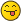Tetration Asymptotic Series - Printable Version +- Tetration Forum (https://tetrationforum.org) +-- Forum: Tetration and Related Topics (https://tetrationforum.org/forumdisplay.php?fid=1) +--- Forum: Mathematical and General Discussion (https://tetrationforum.org/forumdisplay.php?fid=3) +--- Thread: Tetration Asymptotic Series (/showthread.php?tid=1401) Pages: 1 2 Tetration Asymptotic Series - Catullus - 06/08/2022 Sqrt(2) ^^ x is approximated better and better by 2 - a*ln(2)^x + (a^2/(4*(1 - 1/ln(2))))*ln(2)^(2*x), where a is lim x → ∞ (2 - sqrt(2) ^^ x) / ln(2) ^ x. It has an error term of big O of ln(2) ^ 3x. Does anyone know a formula for the asymptotic approximations of a ^^ x when 1 < a < η? Or even more terms, but not a formula for them? All of the higher order terms for b^^x are expressible, in closed form in terms of b's version of a. For base b the analog of a is lim x → ∞ (LambertW(-ln(b))/ln(b)-b^^x)/LambertW(-ln(a))^b. RE: Tetration Asymptotic Series - tommy1729 - 06/08/2022 How did you arrive at that ? RE: Tetration Asymptotic Series - Catullus - 06/09/2022 It was on https://oeis.org/A277435. RE: Tetration Asymptotic Series - bo198214 - 06/09/2022 I think it is based on regular iteration of the formal power series of sqrt(2)^x at fixed point 2. If you look at https://www.researchgate.net/publication/265066072_5_methods_for_real_analytic_tetration chapter 2.3.1 under "Regular C-iteration" you have a similar formula, though the infinite sum is not sorted by powers of s (in your case s=(ln2)^x ) but by powers of z, while in your case z=0 (at the regular iteration of f, not h). I guess after sorting it by powers of s you would arrive at the given formula (and know the further terms). But this really involves quite some understanding and calculation, would be great if someone has some time to explicit it here. RE: Tetration Asymptotic Series - Catullus - 06/09/2022 Hey! You have an icon now. I remember when you did not. RE: Tetration Asymptotic Series - bo198214 - 06/09/2022 (06/09/2022, 09:25 PM)Catullus Wrote: Hey! You have an icon now. I remember when you did not. Yap. RE: Tetration Asymptotic Series - Catullus - 06/10/2022 On the topic of the behavior of tetration. How slow does (e^-e)^^x converge anyway? as n becomes larger and larger (e^-e)^^n approaches e^-1 which is approximately .368. even (e^-e)^^16384~.375. Fun fact: 1/((e^-e)^^16384-e^-1) ~ 141.404. 141.404 ~ sqrt(2)*100. Also maybe there should be a symbol for e^-e. (Also called the iterated exponential constant.) Like how there is a symbol for e^e^-1. RE: Tetration Asymptotic Series - JmsNxn - 06/10/2022 (06/10/2022, 09:56 AM)Catullus Wrote: On the topic of the behavior of tetration. How slow does (e^-e)^^x converge anyway? as n becomes larger and larger (e^-e)^^n approaches e^-1 which is approximately .368. even (e^-e)^^16384~.375. Fun fact: 1/((e^-e)^^16384-e^-1) ~ 141.404. 141.404 ~ sqrt(2)*100. Also maybe there should be a symbol for e^-e. (Also called the iterated exponential constant.) Like how there is a symbol for e^e^-1. I've always thought we should write $$\eta_-$$ for $$e^{-e}$$, as it acts kinda like the polar opposite of $$\eta$$. As to how fast the tetration converges, it is slower than any exponential, and additionally $\sum_{n=0}^\infty \eta_-\uparrow\uparrow n - e^{-1}\,\,\text{Does not converge}\\$ Whereas for every value $$b$$ in the Shellthron region we have exponential convergence to the fixed point. So just hazarding a guess, $$\eta_-\uparrow\uparrow n = e^{-1} + O(1/n)$$. Probably converges slower though, by the amount your showing. I know there is a way to get the correct O term. It's something to do with the petals. I believe there are two petals about $$e^{-e}$$, so if memory serves right, it should be something more like $$O(\sqrt{n})$$. And this aligns with the estimate you have above. So I'd bet it's something like: $\eta_-\uparrow\uparrow n = e^{-1} + O(1/\sqrt{n})$ EDIT: Found it in Milnor. If $$f(z) = e^{2\pi i/k}z + O(z^2)$$, then in the attracting petals (there will be $$k$$), the function $$f^{\circ n}(z) = O(1/\sqrt[k]{n})$$ So yes, an initial estimate would be $$O(\frac{1}{\sqrt{n}})$$. RE: Tetration Asymptotic Series - Catullus - 06/10/2022 (06/10/2022, 08:52 PM)JmsNxn Wrote: I've always thought we should write $$\eta_-$$ for $$e^{-e}$$, as it acts kinda like the polar opposite of $$\eta$$.How about an upside down eta? Quote:So I'd bet it's something like: $\eta_-\uparrow\uparrow n = e^{-1} + O(1/\sqrt{n})$ EDIT: Found it in Milnor. If $$f(z) = e^{2\pi i/k}z + O(z^2)$$, then in the attracting petals (there will be $$k$$), the function $$f^{\circ n}(z) = O(1/\sqrt[k]{n})$$ So yes, an initial estimate would be $$O(\frac{1}{\sqrt{n}})$$. You did not rationalize your denominators. RE: Tetration Asymptotic Series - JmsNxn - 06/10/2022 (06/10/2022, 10:50 PM)Catullus Wrote: (06/10/2022, 08:52 PM)JmsNxn Wrote: I've always thought we should write $$\eta_-$$ for $$e^{-e}$$, as it acts kinda like the polar opposite of $$\eta$$.How about an upside down eta? I love doing things like that, and if I were to write a paper that required rewriting this symbol over and over again, I concur. But mathjax is a stickler for making custom symbols, and it can be a tad annoying to write out the full command everytime you want to say $$e^{-e}$$. But in a paper that would work great (make quick \ueta command). I like your thinking